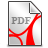# PAI Private Arithmetics Institute

## Programming primitive recursive functions and beyond

The most usual approach to calculative (effectively calculable) functions is done by register machines or similar storage-based computers like the Abacus or Turing machines. Another usual approach to computable functions is to start with primitive recursive functions. However, one has to find a way to put this into a form that does not rely on a pre-knowledge about functions and higher logic. The concrete calcule LAMBDA of decimal pinitive arithmetic allows for such an access. It is based on a machine that is completely different from the storage-based machines: the PINITOR does not use storages but rather many microprocessors, one for each appearance of a command in the code of primitive recursive functior. the codes are decimal numbers, called pinons, where only the characters 0 1 2 8 9 appear. There are just four kind of commands: 0 nullification, 1 succession, 2 straight recursion and 8 composition. The PINITOR is a calculator which means that there is no halting problem. Computers have halting problems, per defintion calculators do not.

Appendix C6 gives the programming of the codes of most of the usual primitive function and goes even farther, e.g. it introduces generator technique that allows for the straight-forward calculation of so-called processive function, that are not primitive recursive. The most famous examples of the Ackermann-function and other hyperexponential functions are programmed.C6-PinonLAMBDA.pdf (579.02KB)C6-PinonLAMBDA.pdf (579.02KB)